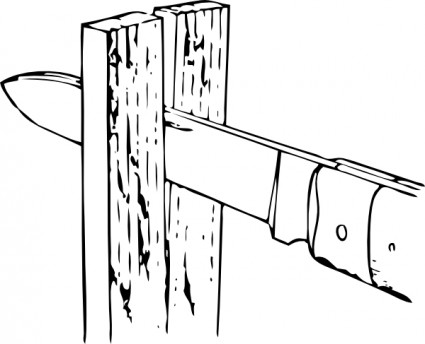# Splitting Into Two PrimesMultiplying two large primes is easy. The result is a huge non-prime number. Factoring this number however, is non-trivial. This is the basis behind a lot of Cryptographic algorithms.

999415083136585001 is a product of two primes. These two primes are palindromes. Find the sum of these two palindromes.

×